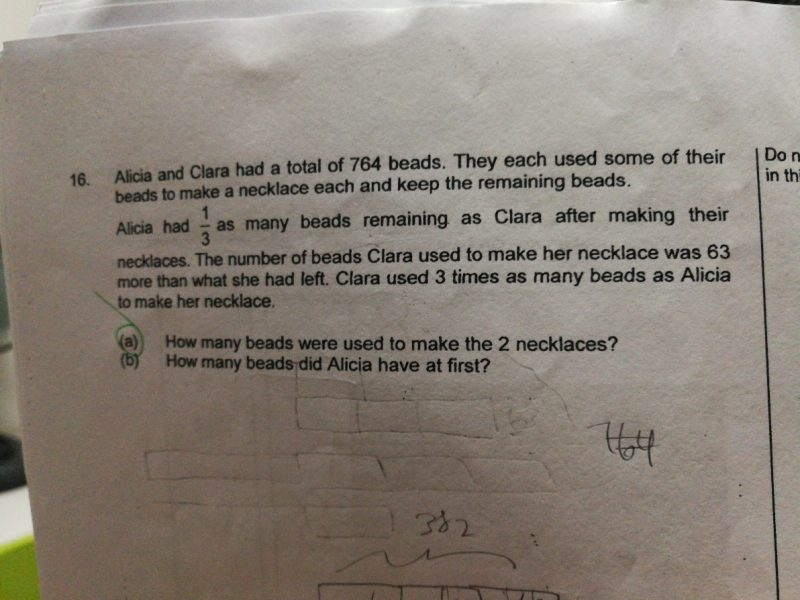# QuestionHi,

Can someone help my DD with the question?

Thank you

1. Remaining:   Alicia = 1/3 of Clara
2. Used:   Clara = 3 Alicia   which is the same as    Alicia = 1/3 Clara
3. Therefore, Initially:  Alicia = 1/3 Clara  also.
4. Initially: Alicia = 764÷(1+3) = 764 ÷ 4 = 191
5. Initially: Clara = 191 x 3 = 573
6. Used: Clara = Remaining + 63 ;
7. therefore, Clara used = (573-63) ÷ 2 +63 = 510 ÷  2 +63 = 255 +63 = 318
8. Alicia used = 318 ÷ 3 = 106

a> Beads used = 106 + 318 = 424

b> Alicia has 191 beads at first

thanks for ur help !

0 Replies 0 Likes

(a)
Alicia left : 1 unit
Clara left : 3 units
Clara used : 3 units + 63
Alicia used : (3 units + 63)/3 = 1 unit + 21
Total : 8 units + 84

8 units + 84 = 764
1 unit = (764 – 84)/8 = 85
3 units + 63 + 1 unit + 21 = 4 units + 84 = 4 x 85 + 84 = 424

(b)
1 unit + 1 unit + 21 = 2 units + 21 = 2 x 85 + 21 = 191